# Homework 6

CS26

80 pts., due Dec. 5

Show all work.

1. (20 pts.) Represent the decimal values 26, -37, 497, and -123 as signed, 10 bit numbers in the following binary formats:
1. Sign-and-magnitude.

2. 1's-complement.

3. 2's-complement.

(See Appendix D for decimal-to-binary conversion.)

2. (20 pts.) Binary fractions are discussed briefly in Section 6.10.
1. Express the decimal values 0.5, -0.123, -0.75, and -0.1 as signed 6-bit numbers in the binary formats of Problem 1.

2. What is the maximum representation error, e, involved in using only 5 significant bits after the binary point?

3. Calculate the number of bits after the binary point so that
1.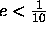2.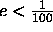3.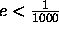4.3. (20 pts.) A half-adder is a combinational logic circuit that has two inputs, x and y, and two outputs, s and c, that are the sum and carry-out, respectively, resulting from the binary addition of x and y.
1. Design a half-adder as a two-level AND-OR circuit.

2. Show how to implement a full-adder, as shown in Figure 6.4a, by using two half-adders and external logic gates.

3. Compare the longest path logic delay through the network derived in part (b) to that of the logic delay of the adder network shown in Figure 6.4a.

4. (20 pts.) 16 of the 4-bit adder circuits shown in Figure 6.7 can be cascaded to form a 64-bit adder. In this cascade, the output,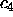, from the low-order circuit is connected as the carry-in to the next circuit. Its carry-out,, is connected to the carry-in of the third circuit, and so on. The circuit has 129 inputs (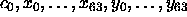) and 65 outputs (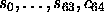).
1. Draw the cascaded circuit. Using the delay model developed in class, determine the worst-case number of gate delays through the circuit.

2. Using 4-bit adder circuits and 4-bit carry lookahead units, design a 64-bit full carry lookahead adder. Draw the circuit. Again, using the delay model developed in class, determine the worst-case number of gate delays through the circuit.

3. Compare the delays. Is the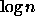speed-up of the full carry lookahead adder valid for arbitrarily large values of n? Why or why not?

Thomas P. Kelliher
Mon Nov 25 10:31:19 EST 1996
Tom Kelliher